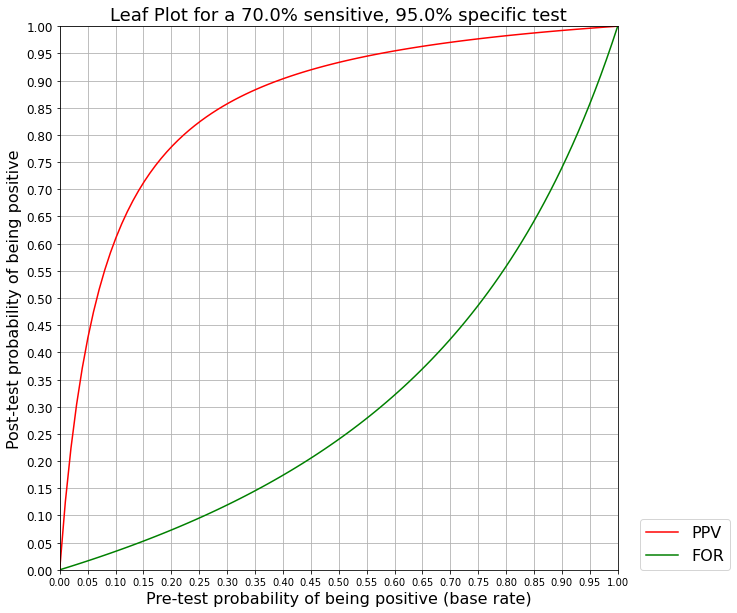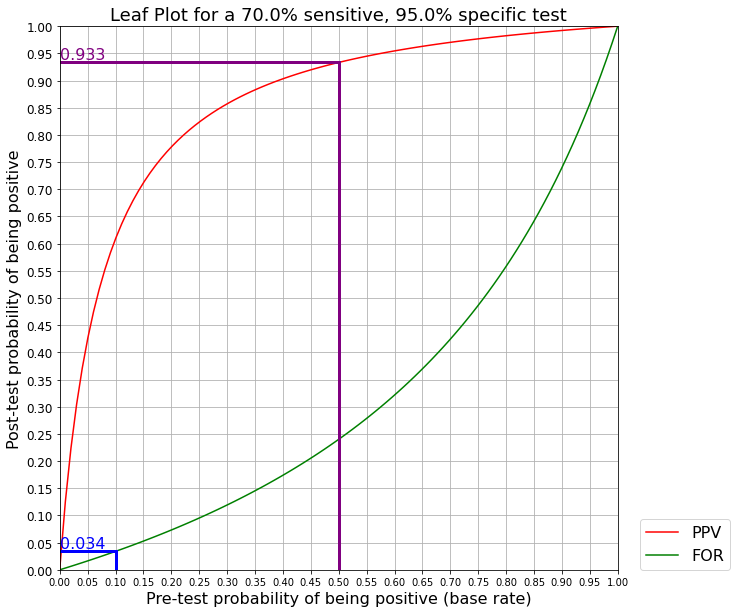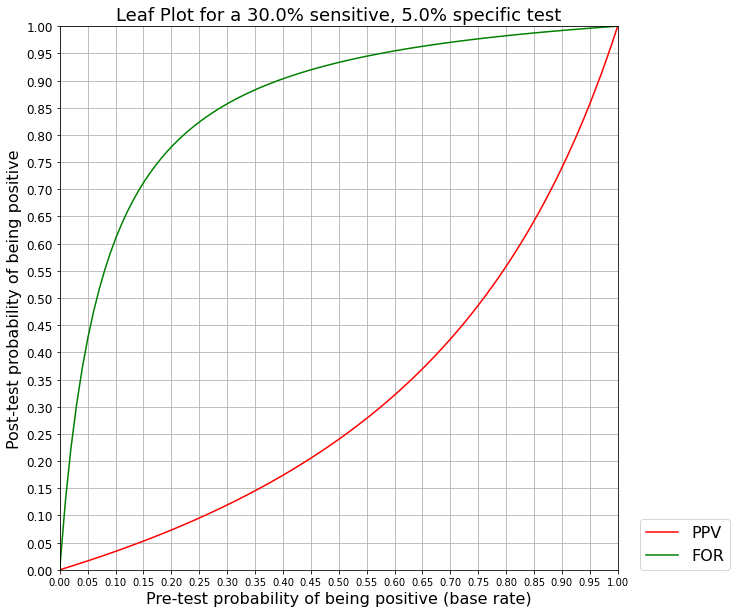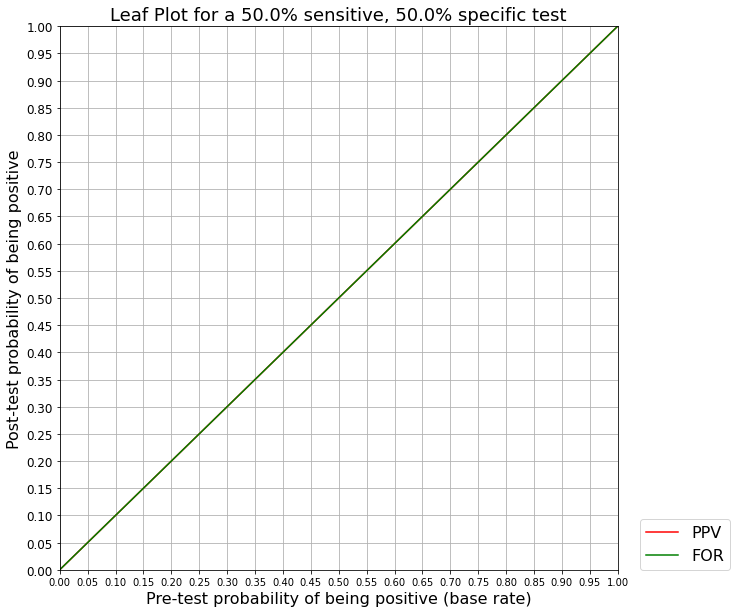# Leaf Plots for interpreting test results

This is a follow-up to my previous article about interpreting test results and the base rate fallacy. Since I wrote that, I read about a straightforward way to visualize the relationship between pre and post-test probability, called leaf plots. Here, I’ll give my overview of them, which is also available as a Jupyter Notebook.

Test performance is usually measured in terms of the sensitivity and specificity, which are the True Positive Rate (TPR) and True Negative Rate (TNR) respectively. They are defined as:

• Sensitivity: The proportion of positives correctly identified as positive. For example, if there were 100 positives but the test only identified 90 of them as positive, the sensitivity would be 0.90.
• Specificity: The proportion of negatives correctly identified as negative. For example, if there were 50 negatives but the test only identified 40 of them as negative, the specificity would be 0.80.

It’s not straightforward to understand how sensitivity and specificity translate into answering the question: What proportion of people who test positive will actually have the condition? This is known as the Positive Predictive Value (PPV) or the Precision of the test, and it depends not only on the sensitivity and specificity but also the base rate of the condition in the population being tested. This base rate can also be thought of as the pre-test probability.

Additionally, you may be concerned with what proportion of people who test negative actually turn out to have the condition. This is called the False Omission Rate (FOR), and it also depends on the sensitivity, specificity, and the pre-test probability.

However, even if you understand how to calculate the PPV and FOR it’s often difficult to gain an intuitive understanding of how to interpret the result of a positive or negative test. Having a visual aid can help.

# Enter Leaf Plots

In “The leaf plot: a novel way of presenting the value of tests” (MG Coulthard, 2019), leaf plots are proposed as a way of making the interpretation of a test result (positive or negative) easier in determining whether the patient actually has the condition or not.

We can think of this in an informal Bayesian context. The pre-test probability of the condition is our prior, and the test result (positive or negative) is our data that when combined with the knowledge of the test’s sensitivity and specificity, transforms the pre-test probability into a post-test probability or posterior.

Let’s take a look at these leaf plots and discuss their usage.

# Interpreting a Leaf Plot

A leaf plot consists of two curves:

1. The PPV as a function of the pre-test probability.
2. The FOR as a function of the pre-test probability.

The two curves form a leaf-like figure, giving rise to the name. Here is an example of a leaf plot:A leaf plot

We can use a leaf plot to determine the post-test probability of being positive given a test result and the pre-test probability in the following way:

• If the test result is positive, then the post-test probability of being positive is the corresponding point on the PPV curve.
• For example in the above leaf plot, if we have a pre-test probability of 0.10, then a positive test yields a post-test probability of ~0.61. In other words, there is only a 61% chance that a positive result really means you are positive.
• If the test result is negative, then the post-test probability of being positive is the corresponding point on the FOR curve.
• For example in the above leave plot, if we have a base rate of 0.10, then a negative test yields a post-test probability of ~0.034. In other words, a negative test result means there is still a 3.4% chance of being positive.

This can be easier to see if we draw horizontal and vertical lines on the chart. If we draw a vertical line at the value of the pre-test probability, it will intersect both the PPV and the FOR curves. We can then draw a horizontal line from this intersection point over to the left-hand side of the graph, and read off the associated post-test probability.

The following graph illustrates this:A leaf plot with separate positive and negative test results

In the above graph, the BLUE line represents a negative test result when the pre-test probability (base rate) is 10%. In that case, we go UP from 0.10 on the x-axis until we hit the FOR curve, and then over to the LEFT to read off the post-test probability, which is ~3.4% in this case.

The PURPLE line represents a positive test result when the pre-test probabilty is 50%. We go UP from 0.50 on the x-axis until we hit the PPV curve, and then over to the LEFT to read off the post-test probability, which is ~93.3%.

# Implications

A leaf plot helps to visualize the post test-probability as a function of the sensitivity and specificity of a test, and the pre-test probability.

For example, for our 70% sensitive and 95% specific test, if the pre-test probability is only 5% (0.05), then only 42% of people who test positive will actually be positive! This means many positive tests under these conditions will be false positives, and thus a positive test here is not a reliable indicator. (By contrast, only 1.6% of people who test negative will actually be positive, meaning a negative test here is a reliable indicator.) If we use this test to screen a population where the prevalence of the condition is expected to be low, we can expect many false positives.

However, if the pre-test probability is considerably higher at 50%, then we have the reverse problem: Many people who test negative will actually be positive. Under these conditions, 24% of people who test negative will actually be positive, so a negative test cannot be reliably used to exclude people from having the condition! This is important if the condition is a disease that is contagious. (By contrast, 93.3% of people who test positive here will be actually positive, so a positive test here is a reliable indicator)

The leaf plot helps us visualize how important the pre-test probability is in determining the fraction of false positives or false negatives a test will generate.

All else being equal, as the pre-test probability rises, the PPV also rises, which means that the value of a positive test goes up, and you can be more sure that a positive test really means the condition is present. This is the reason why we generally don’t screen healthy people with no symptoms with tests for various diseases - the pre-test probability might be too low relative to the sensitivity/specificity of the test for a positive result to be meaningful.

But at the same time, as the pre-test probability rises, so does the FOR. This means the probability of a negative test result being wrong, and the condition actually being present, increases. If we are using a test on someone who is displaying many symptoms associated with the condition we are testing for, a negative test result may not be enough to exclude them from having the condition.

# Other properties of the leaf plot

In a leaf plot of any real test, the PPV should always be above or equal to the FOR. Intuitively, this makes sense: Testing negative should never result in a higher probability of having the condition than testing positive!

If the PPV is below the FOR, this means the test is so bad that you should interpret the opposite of what it outputs. That is, you should treat all positive results as negatives, and all negative results as positives, and the test would actually do better.

Here is an example of a hypothetically bad test with a sensitivity of 30% and a specificity of 5%. With such poor results, the PPV curve is actually below the FOR curve.A leaf plot for a hypothetically bad test

Recall that sensitivity is the true positive rate, or the fraction of positives identified as positive by the test. If the sensitivity was only 30%, that means the test incorrectly identified 70% of positives as being negative. If we just flipped things around, and treated the negative results as positives, then the sensitivity would be increased to 70%, which is simply the complement of 30%.

The same reasoning applies for the specificity (true negative rate).

Because of this, the worst a test can do is have a sensitivity of 50% and a specificity of 50%. This is equivalent to flipping a coin to decide what the test result should be. A leaf plot of this test shows that the PPV and FOR overlap, and they are both the line $y = x$:Leaf plot for a coin flip test

Such a test is completely useless, because whatever the pre-test probability is, the post-test probability is always the same value because both the PPV and FOR curves are the line $y = x$. That is, doing the test doesn’t affect the probability of whether someone has the condition or not, and thus the test provides no information.

1. The leaf plot: a novel way of presenting the value of tests: https://www.ncbi.nlm.nih.gov/pmc/articles/PMC6428474/
2. Interpreting a covid-19 test result: https://www.bmj.com/content/bmj/369/bmj.m1808.full.pdf
3. Interpreting a covid-19 test result (Interactive Visualization): https://www.bmj.com/content/369/bmj.m1808
4. https://en.wikipedia.org/wiki/Sensitivity_and_specificity
5. https://en.wikipedia.org/wiki/Positive_and_negative_predictive_values